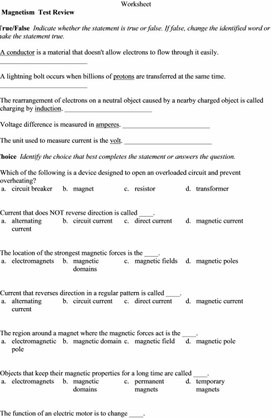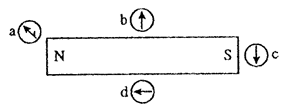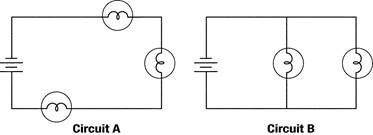PHY_10_65_V2_DM_Electromagnetic devices
Оценка 5

# PHY_10_65_V2_DM_Electromagnetic devices

Оценка 5
docx
08.05.2020PHY_10_65_V2_DM_Electromagnetic devices.docx

Worksheet

Electricity Magnetism  Test Review

Modified True/False  Indicate whether the statement is true or false. If false, change the identified word or phrase to make the statement true.

1.   A conductor is a material that doesn't allow electrons to flow through it easily. _________________________

2.   A lightning bolt occurs when billions of protons are transferred at the same time. _________________________

3.   The rearrangement of electrons on a neutral object caused by a nearby charged object is called charging by induction. _________________________

4.   Voltage difference is measured in amperes. _________________________

5.   The unit used to measure current is the volt. _________________________

Multiple Choice  Identify the choice that best completes the statement or answers the question.

6.   Which of the following is a device designed to open an overloaded circuit and prevent overheating?

 a. circuit breaker b. magnet c. resistor d. transformer

7.   Current that does NOT reverse direction is called ____.

 a. alternating current b. circuit current c. direct current d. magnetic current

8.   The location of the strongest magnetic forces is the ____.

 a. electromagnets b. magnetic domains c. magnetic fields d. magnetic poles

9.   Current that reverses direction in a regular pattern is called ____.

 a. alternating current b. circuit current c. direct current d. magnetic current

10.   The region around a magnet where the magnetic forces act is the ____.

 a. electromagnetic pole b. magnetic domain c. magnetic field d. magnetic pole

11.   Objects that keep their magnetic properties for a long time are called ____.

 a. electromagnets b. magnetic domains c. permanent magnets d. temporary magnets

12.   The function of an electric motor is to change ____.

 a. chemical energy to electrical energy c. electrical energy to mechanical energy b. electrical energy to chemical energy d. mechanical energy to electrical energy

13.   The function of a generator is to change ____.

 a. chemical energy to electrical energy c. electrical energy to mechanical energy b. electrical energy to chemical energy d. mechanical energy to electrical energy

14.   The current that flows in an electric circuit carries ____.

 a. chemical energy b. mechanical energy c. thermal energy d. electrical energy

15.   There is a repulsive force between two charged objects when

 a. charges are of unlike sign. c. charges are of like sign. b. they have the same number of protons. d. they have the same number of electrons.

16.   There is an attractive force between two charged objects when

 a. charges are of unlike sign. c. charges are of like sign. b. they have the same number of protons. d. they have the same number of electrons.17.   Which of the compass needle orientations in the figure above might correctly describe the magnet’s field at that point?

 a. a b. b c. c d. d

Matching

Match each term with the correct statement below.

 a. conductor d. fuse b. insulator e. electroscope c. circuit breaker

____  18.   contains a piece of metal that melts if the current becomes too high

____  19.   allows electrons to move through it easily

____  20.   contains a piece of metal that bends when it gets hot

____  21.   detects the presence of electric charges

____  22.   does not allow electrons to move through it easily

Completion   Complete each statement.Figure 7-1

23.   In Figure 7-1, circuit ____________________ is wired in series.

24.   In Figure 7-1, circuit ____________________ is wired in parallel.

25.   In Figure 7-1, circuit ____________________ represents the way that homes are usually wired.

26.   In Figure 7-1, circuit ____________________ is the type of circuit that causes an entire string of decorative lights to go out when one of the bulbs burns out.

Скачано с www.znanio.ru

Скачать файл